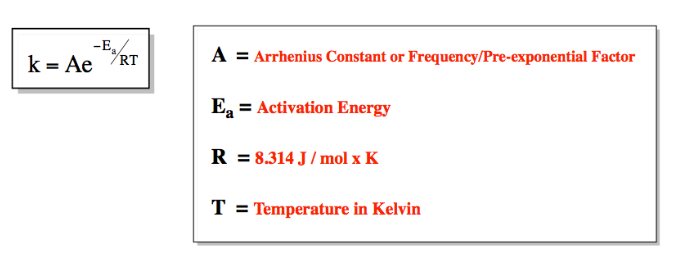# Problem: A popular chemical demonstration is the “magic genie” procedure, in which hydrogen peroxide decomposes to water and oxygen gas with the aid of a catalyst. The activation energy of this (uncatalyzed) reaction is 70.0 kJ/mol. When the catalyst is added, the activation energy (at 20.°C) is 42.0 kJ/mol. Theoretically, to what temperature (°C) would one have to heat the hydrogen peroxide solution so that the rate of the uncatalyzed reaction is equal to the rate of the catalyzed reaction at 20.°C? Assume the frequency factor A is constant, and assume the initial concentrations are the same.

###### FREE Expert Solution

The Arrhenius Equation shows the dependence of the rate constant on the pre-exponential factor, absolute temperature and activation energy of the reaction.99% (102 ratings)###### Problem Details

A popular chemical demonstration is the “magic genie” procedure, in which hydrogen peroxide decomposes to water and oxygen gas with the aid of a catalyst. The activation energy of this (uncatalyzed) reaction is 70.0 kJ/mol. When the catalyst is added, the activation energy (at 20.°C) is 42.0 kJ/mol. Theoretically, to what temperature (°C) would one have to heat the hydrogen peroxide solution so that the rate of the uncatalyzed reaction is equal to the rate of the catalyzed reaction at 20.°C? Assume the frequency factor A is constant, and assume the initial concentrations are the same.